# Using a Range Name in Formula in Microsoft Excel

In this article, we will learn how we can use defined range name while calculation in Microsoft Excel.

Let’s take an example to understand.

We have a workbook in which we have 3 lists in 3 columns. Column A contains Jan_2010 sold number to the product, same column B contains the number for Feb_2010, and column C contains the number for the month of Mar_2010.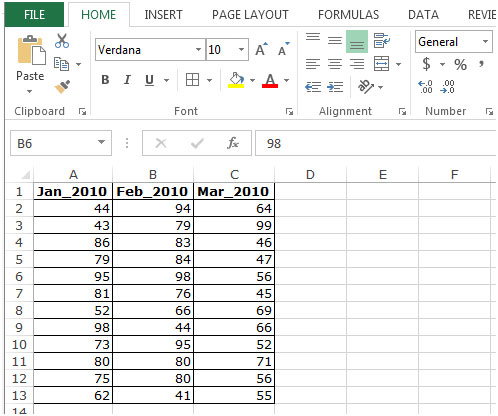Follow below given steps to use a range name in a formula:-

• Define the name to every column.
• Select the range A1:A13, and define the name in Name box.
• Write Jan_2010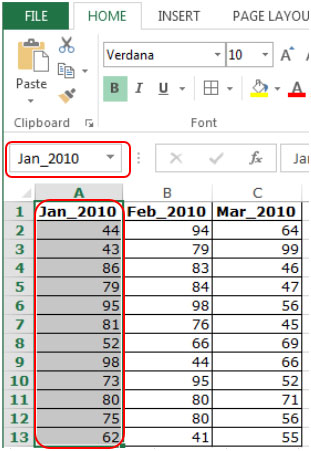• Select the range B1:B13, and define the name in Name box.
• Write Feb_2010.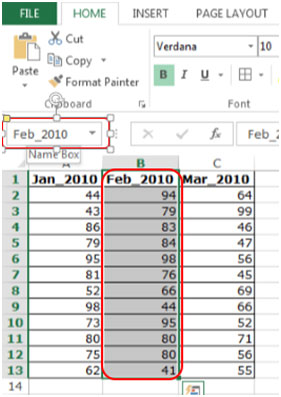• Select the range C1:C13, and define the name in Name box.
• Write Mar_2010.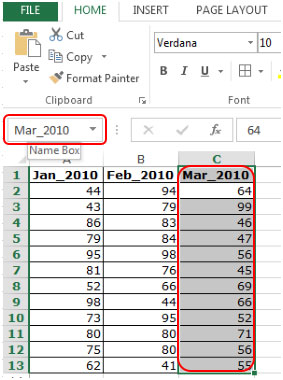• Select a cell and type the formula.
• =Sum(Jan_2010,Feb_2010,_Mar_2010).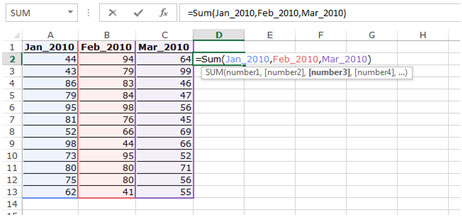• Press Enter on your keyboard.
• The function will sum the values to the defined range.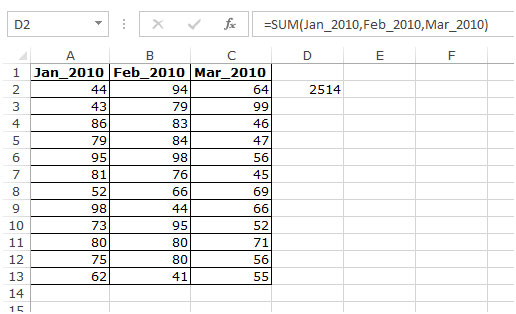This is the way we can use the formula to define a range name in Microsoft Excel.

Terms and Conditions of use

The applications/code on this site are distributed as is and without warranties or liability. In no event shall the owner of the copyrights, or the authors of the applications/code be liable for any loss of profit, any problems or any damage resulting from the use or evaluation of the applications/code.